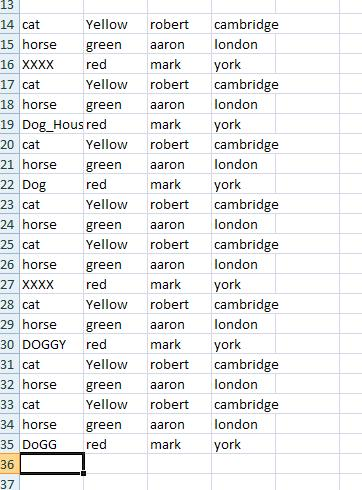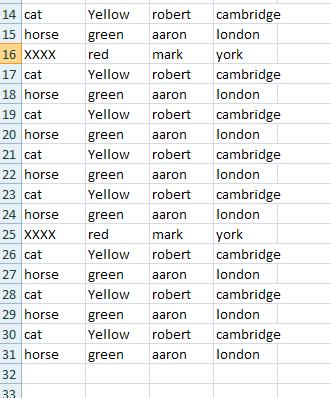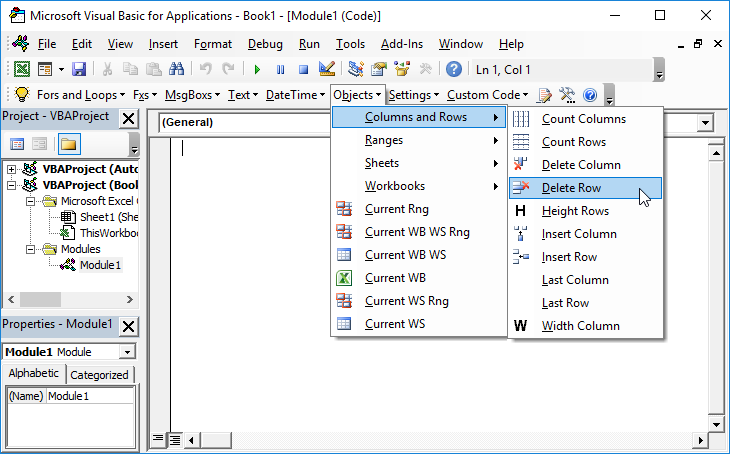### Delete Rows that Meet Certain Criteria in VBA

The following Subroutine will delete each row in a range where the value in Column A begins with a prescribed piece of text:

```Sub Delete_Rows(Data_range As Range, Text As String)
Dim Row_Counter As Integer
For Row_Counter = Data_range.Rows.Count To 1 Step -1
If Data_range Is Nothing Then

Exit Sub
End If
If UCase(Left(Data_range.Cells(Row_Counter, 1).Value, Len(Text))) = UCase(Text) Then
Data_range.Cells(Row_Counter, 1).EntireRow.Delete
End If
Next Row_Counter

End Sub```

For example Delete_Rows(Sheets(“Sheet1”).Range(“A1:E23”,”Dog”) will delete all the rows in the range A1:E23 where the value in Column A begins with the word “Dog”. Note the use of Ucase means that the formulae is case INSENSITIVE i.e cells that begin with any of DOG, Dog, DoG or dog will all be deleted.

This:Will become: